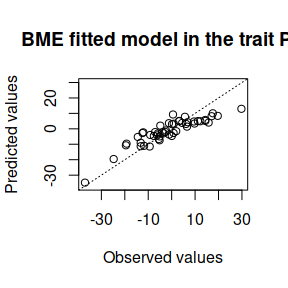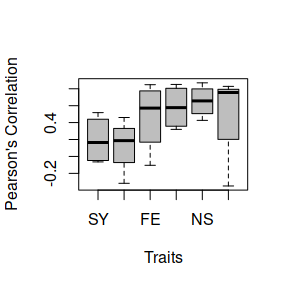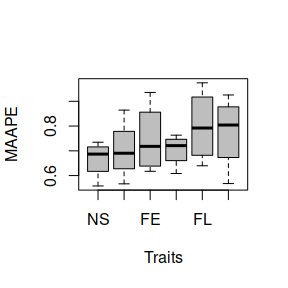# Fit the Bayesian Multi-Environment model and apply a random partition cross-validation

#### 2019-02-11

This example illustrates how to fit a model where there is only one environment and several traits, even though it may contradict the model. To do this, the WheatMadaToy data set is used. To load the data set, the data() function as shown below:

library(BMTME)
data('WheatMadaToy')

With the command, the following 2 objects are loaded into the R environment:

• genoMada: Genomic matrix of the data set.
• phenoMada: Phenotypic data from the data set.

The phenotypic data has the following structure:

GID PH FL FE NS SY NP
9 29.7776 -8.8882 -4.93900 1.04100 169.06 28.8025
11 3.2210 -7.1111 -0.36940 -3.88940 -107.19 58.2516
12 6.1670 -9.5337 -12.43680 2.58250 -160.54 17.1278
15 6.8117 4.6377 11.78860 -0.03378 235.70 -19.6571
20 -14.4480 3.2525 6.40780 -14.23460 131.87 42.2962
21 -13.2185 3.8902 0.09722 5.35680 164.06 36.8239

Then we proceed to define the model to be adjusted, as the data set only includes an environment where several traits were evaluated, the BME model is used, and for its implementation, first we need order the dataset as follows:

phenoMada <- (phenoMada[order(phenoMada$GID),]) This is the most important step in the analysis, because if the dataset is not ordered by the identifiers, may cause conflicts between the analysis producing incorrect estimations. Then, the matrix design for the genetic effects should be generated, as shown below, LG <- cholesky(genoMada) ZG <- model.matrix(~0 + as.factor(phenoMada$GID))
Z.G <- ZG %*% LG

Then, we extract the phenotypic responses and it’s converted to matrix object as shown in the following command,

Y <- as.matrix(phenoMada[, -c(1)])

Finally, the model is adjusted, and for demonstration purposes only 1250 iterations are used to adjust the model.

fm <- BME(Y = Y, Z1 = Z.G, nIter = 1250, burnIn = 500, thin = 2, bs = 50)
fm
#> Multi-Environment Model Fitted with:
#>  1250  Iterations, burning the first  500  and thining every  2
#>  We found  0  NA values
#>
#>
#>  Use str() function to found more detailed information.

To know all the details about the output of the fitted model, we use the str() function, which returns the following information:

str(fm)
#> List of 17
#>  $Y : num [1:50, 1:6] 29.78 3.22 6.17 6.81 -14.45 ... #> ..- attr(*, "dimnames")=List of 2 #> .. ..$ : chr [1:50] "1" "2" "3" "4" ...
#>   .. ..$: chr [1:6] "PH" "FL" "FE" "NS" ... #>$ nIter      : num 1250
#>  $burnIn : num 500 #>$ thin       : num 2
#>  $dfe : int 5 #>$ Se         : num [1:6, 1:6] 527.9 -29.6 28.3 74.3 1523.2 ...
#>   ..- attr(*, "dimnames")=List of 2
#>   .. ..$: chr [1:6] "PH" "FL" "FE" "NS" ... #> .. ..$ : chr [1:6] "PH" "FL" "FE" "NS" ...
#>  $yHat : num [1:50, 1:6] 12.97 5.1 3.14 4.28 -5.27 ... #> ..- attr(*, "dimnames")=List of 2 #> .. ..$ : NULL
#>   .. ..$: chr [1:6] "PH" "FL" "FE" "NS" ... #>$ SD.yHat    : num [1:50, 1:6] 5.98 4.37 4.34 3.55 5.02 ...
#>   ..- attr(*, "dimnames")=List of 2
#>   .. ..$: NULL #> .. ..$ : chr [1:6] "PH" "FL" "FE" "NS" ...
#>  $beta : num [1, 1:6] -1.0723 0.0233 2.3083 0.4236 -15.3493 ... #> ..- attr(*, "dimnames")=List of 2 #> .. ..$ : NULL
#>   .. ..$: chr [1:6] "PH" "FL" "FE" "NS" ... #>$ SD.beta    : num [1, 1:6] 0.9171 0.4605 0.883 0.9529 0.0793 ...
#>   ..- attr(*, "dimnames")=List of 2
#>   .. ..$: NULL #> .. ..$ : chr [1:6] "PH" "FL" "FE" "NS" ...
#>  $b1 : num [1:50, 1:6] -11.38 12.81 1.96 5.77 -7.45 ... #> ..- attr(*, "dimnames")=List of 2 #> .. ..$ : NULL
#>   .. ..$: chr [1:6] "PH" "FL" "FE" "NS" ... #>$ SD.b1      : num [1:50, 1:6] 2.18 3.49 3.45 3.6 4.29 ...
#>   ..- attr(*, "dimnames")=List of 2
#>   .. ..$: NULL #> .. ..$ : chr [1:6] "PH" "FL" "FE" "NS" ...
#>  $vare : num [1:6, 1:6] 66.58 -2.33 -11.3 20.02 903.56 ... #> ..- attr(*, "dimnames")=List of 2 #> .. ..$ : NULL
#>   .. ..$: chr [1:6] "PH" "FL" "FE" "NS" ... #>$ SD.vare    : num [1:6, 1:6] 18.28 4.89 9.43 12.17 401.21 ...
#>   ..- attr(*, "dimnames")=List of 2
#>   .. ..$: NULL #> .. ..$ : chr [1:6] "PH" "FL" "FE" "NS" ...
#>  $varTrait : num [1:6, 1:6] 64.14 -4.45 4.66 1.5 -87.68 ... #> ..- attr(*, "dimnames")=List of 2 #> .. ..$ : NULL
#>   .. ..$: chr [1:6] "PH" "FL" "FE" "NS" ... #>$ SD.varTrait: num [1:6, 1:6] 22.53 5.59 10.98 14.53 382.25 ...
#>   ..- attr(*, "dimnames")=List of 2
#>   .. ..$: NULL #> .. ..$ : chr [1:6] "PH" "FL" "FE" "NS" ...
#>  $NAvalues : int 0 #> - attr(*, "class")= chr "BME" Where we can observe that the returned data corresponds to the observed values ($Y), the parameters provided for the model fit ($nIter, $burnIn, $thin, etc.), the predicted values ($yHat) and the estimations of the beta coefficients, random effects of lines and the genetic and residual covariances ($beta, $SD.beta, $b1, $SD.b1, $varTrait, $vare, etc.). Since breeders are interested in understanding the matrix of covariance and genetic correlation, these can be extracted with the following command,

COV_TraitGenetic <- fm$varTrait COV_TraitGenetic #> PH FL FE NS SY NP #> [1,] 64.1411 -4.4492 4.6643 1.5014 -87.6812 -103.1898 #> [2,] -4.4492 6.4304 3.7380 -3.2814 157.7148 -16.6803 #> [3,] 4.6643 3.7380 22.4609 -3.1348 -124.0044 -37.2303 #> [4,] 1.5014 -3.2814 -3.1348 49.5229 267.1689 -67.0150 #> [5,] -87.6812 157.7148 -124.0044 267.1689 24520.3893 827.4957 #> [6,] -103.1898 -16.6803 -37.2303 -67.0150 827.4957 877.1993 To convert this covariance matrix into a correlation matrix it is suggested to use the following command, COR_TraitGenetic <- cov2cor(COV_TraitGenetic) COR_TraitGenetic #> PH FL FE NS SY NP #> [1,] 1.00000000 -0.2190760 0.12288665 0.02663945 -0.06991568 -0.4350302 #> [2,] -0.21907596 1.0000000 0.31103318 -0.18388112 0.39718220 -0.2220934 #> [3,] 0.12288665 0.3110332 1.00000000 -0.09399251 -0.16709361 -0.2652368 #> [4,] 0.02663945 -0.1838811 -0.09399251 1.00000000 0.24244832 -0.3215288 #> [5,] -0.06991568 0.3971822 -0.16709361 0.24244832 1.00000000 0.1784239 #> [6,] -0.43503022 -0.2220934 -0.26523682 -0.32152878 0.17842386 1.0000000 Where we can observe that there is not a high correlation (greater than 0.5). Below is a sample of how we can obtain the residual covariance (correlation) matrix, COV_ResGenetic <- fm$vare
COV_ResGenetic
#>            PH       FL        FE       NS         SY        NP
#> [1,]  66.5753  -2.3303  -11.3049  20.0187   903.5584  -80.0744
#> [2,]  -2.3303  11.2498   10.0587  -6.1940   194.9712  -11.9277
#> [3,] -11.3049  10.0587   39.2669 -18.0235  -428.3902  -32.7667
#> [4,]  20.0187  -6.1940  -18.0235  53.2206   426.5239  -69.8469
#> [5,] 903.5584 194.9712 -428.3902 426.5239 61402.5764 1813.5992
#> [6,] -80.0744 -11.9277  -32.7667 -69.8469  1813.5992  682.1164

To convert the residual matrix into the correlation matrix it is suggested to use the following command,

COR_ResGenetic <- cov2cor(COV_ResGenetic)
COR_ResGenetic
#>               PH          FL         FE         NS         SY         NP
#> [1,]  1.00000000 -0.08514965 -0.2211041  0.3363097  0.4468959 -0.3757577
#> [2,] -0.08514965  1.00000000  0.4785818 -0.2531389  0.2345874 -0.1361617
#> [3,] -0.22110413  0.47858176  1.0000000 -0.3942629 -0.2758881 -0.2002120
#> [4,]  0.33630972 -0.25313892 -0.3942629  1.0000000  0.2359447 -0.3665878
#> [5,]  0.44689589  0.23458745 -0.2758881  0.2359447  1.0000000  0.2802327
#> [6,] -0.37575769 -0.13616173 -0.2002120 -0.3665878  0.2802327  1.0000000

Where we can observe that the residual of traits is not highly correlated (greater than 0.5). On the other hand, to extract the predicted values from the model it is necessary call the $yhat values of the object within the adjusted model, for demonstration purposes we will only extract the first 6 predictions for the 6 traits evaluated. head(fm$yHat)
#>           PH      FL     FE      NS        SY       NP
#> [1,] 12.9661 -4.7013 2.1153 -4.7628 -157.3326  31.0674
#> [2,]  5.1025 -2.4873 2.4544 -2.7864  -69.7211  27.0256
#> [3,]  3.1409 -1.9228 0.5203 -1.7902  -84.1670   8.6228
#> [4,]  4.2808  1.8776 5.4652  0.5422   56.8625 -18.1802
#> [5,] -5.2653  1.2219 2.5633 -3.9485   49.7080  13.1858
#> [6,] -9.0255  1.1104 0.8005  3.4156   68.4979  24.2124

The software also allows plotting the observed values against the predicted values by trait, as shown below:

plot(fm, trait = 'PH')## Cross validation with random partitions

On the other hand, the package also allows making cross-validation for the predictions for this we require a data.frame object with the phenotypes as illustrated below:

pheno <- data.frame(GID = phenoMada[, 1], Env = '', Response = phenoMada[, 3])

Once the object is generated, we will use the CV.RandomPart() function, to form the training and testing sets of each random partition of the cross validation, it is suggested to provide the number of random partitions, the percentage of the data to be used for the testing and a seed to guarantee a reproducible analysis.

CrossV <- CV.RandomPart(pheno, NPartitions = 4, PTesting = 0.2, set_seed = 123)

Since the partitions have been generated for cross validation, we will use the BME() function to fit the model, however, we will include the above object in the testingSet parameter to implement cross validation. In addition, we will use the summary() function, to show the resulting predictive capacity by trait evaluated.

pm <- BME(Y = Y, Z1 = Z.G, nIter = 1000, burnIn = 500, thin = 2, bs = 50,
testingSet = CrossV, progressBar = FALSE)
summary(pm)
#>   Environment Trait Pearson SE_Pearson  MAAPE SE_MAAPE
#> 1                FE  0.4715     0.2096 0.7472   0.0714
#> 2                FL  0.1293     0.1624 0.7996   0.0739
#> 3                NP  0.4975     0.2827 0.7033   0.0334
#> 4                NS  0.6522     0.0950 0.6664   0.0382
#> 5                PH  0.5811     0.1315 0.7754   0.0757
#> 6                SY  0.1956     0.1446 0.7029   0.0613

We can observe that the results are given around the traits that have been evaluated, showing for each of them the predictive capacity obtained using the Pearson correlation average of all the partitions used in the cross validation; as well as the mean arctangent percentage error (MAAPE). From the results obtained, we can emphasize that the traits that have obtained a better predictive capacity were the NS and PH traits. While the SY and FL traits have low predictive capabilities. In addition, the package offers the ability to generate graphs of the results obtained, for it we will use the function that appears next to show a boxplot with the results obtained.

boxplot(pm)In this figure we can observe that the PH trait has obtained the best predictive capacity on average, while the SY trait has obtained the lowest predictive capacity. It is also possible to graph the predictive capacity of the traits under study using the MAAPE, just specify it through the select parameter, as shown below,

boxplot(pm, select = 'MAAPE')In this figure we can observe that the FL trait has been the one that has obtained the highest MAAPE index and therefore presents the worst prediction, but it is like the rest of them, so we cannot trust in the predictions of the analysis.